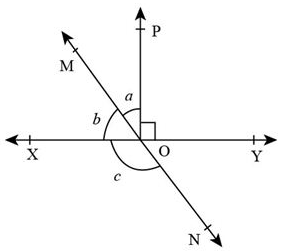QUESTION

# In the given figure, lines XY and MN intersect at O. If $\angle POY = {90^ \circ }$ and a:b = 2 : 3, then $\angle XON$ is equal to……..A. ${126^ \circ }$B. ${30^ \circ }$C. ${90^ \circ }$D. ${180^ \circ }$

Hint:For solving this question, we use the concept of linear pair of angles. We will use axiom1 of linear pair of angles for finding out the value of a and b. As given a:b = 2:3, a = 2x, b = 3x, adding a and b and $\angle POY$sum should be equal to ${180^ \circ }$. Similarly applying axiom1 on b and c we will be able to find the value of angle $\angle XON$.

Linear pair of angles: If non common arms of two adjacent angles form a line, then these angles are called linear pairs of angles. There are basically two axioms for linear pair of angles known as linear pair axioms they are as follow:
Axiom1: If a ray stands on a line, then the sum of two adjacent angles so formed is 180°i.e, the sum of the linear pair is 180°. Axiom2: If the sum of two adjacent angles is 180° then the two non common arms of the angles form a line.Given,
$\angle POY = {90^ \circ }\,\,\,\,\,\, \ldots ..{\text{ }}\left( 1 \right)$
$a:b = 2:3\,\,\,\,\,\,\, \ldots ..{\text{ }}\left( 2 \right)$
Let the common ratio between a and b be x, therefore, a = 2x, b = 3x.
According to the question: $\angle POY + \angle POX = {180^ \circ }\,\,\,\,\,\,\, \ldots ..{\text{ }}\left( 3 \right)$ [By linear pair axiom]
Given, $\angle POX = a + b\,\,\,\,\, \ldots ..{\text{ }}\left( 4 \right)$
Substituting equation (4) in equation (3), we get,
$\Rightarrow \angle POY\; + {\text{ }}a{\text{ }} + {\text{ }}b{\text{ }} = {\text{ }}{180^ \circ }\,\,\,\,\, \ldots ..{\text{ }}\left( 5 \right)$
Substituting equation (1) in equation (5), we get,
$\Rightarrow 90^\circ + {\text{ }}a{\text{ }} + {\text{ }}b{\text{ }} = {\text{ }}{180^ \circ }$
$\Rightarrow a{\text{ }} + {\text{ }}b{\text{ }} = {\text{ }}{90^ \circ }$ $.....(6)$
Substituting value of a = 2x and b =3x in equation (6), we get,
$\begin{array}{*{20}{l}} { \Rightarrow 5x{\text{ }} = {\text{ }}{{90}^ \circ }} \\ { \Rightarrow x{\text{ }} = {\text{ }}{{18}^ \circ }} \end{array}$
Therefore,
$\Rightarrow a = 2x = 2({18^ \circ }) = {36^ \circ }$
$\Rightarrow b = 3x = 3({18^ \circ }) = {54^ \circ }\,\,\,\,\,\,\,\,\,\,\,\,\,\,\,\,\,\,\,\,\,.....\,(7)$
Now, OX is a ray on the line MON.
$\angle XOM{\text{ }} + \angle XON = {180^ \circ }$
$\angle XOM = b,\,\angle XON = c$
$b{\text{ }} + {\text{ }}c{\text{ }} = {\text{ }}{180^ \circ }\,\,\,\,\,\,\,\,\,\,.....(8)$ (by Linear Pair axiom)
Substituting equation (7) in equation (8), we get,
$\begin{array}{*{20}{l}} { \Rightarrow {{54}^ \circ } + c = {{180}^ \circ }} \\ { \Rightarrow c = {{126}^ \circ }} \end{array}$
Therefore, the value of c = ${126^ \circ }$
Hence, the correct answer is option (A.) ${126^ \circ }$.

Note: The most common mistake while solving this type of questions, occurs when we calculate angles using axioms of linear pair of angles. Another method to find out the value of $\angle XON$ is by adding ${90^ \circ }$ to a.